# Basics of Functions Chapter Exam

Exam Instructions:

Choose your answers to the questions and click 'Next' to see the next set of questions. You can skip questions if you would like and come back to them later with the yellow "Go To First Skipped Question" button. When you have completed the practice exam, a green submit button will appear. Click it to see your results. Good luck!

### Page 2

#### Question 8 8. What are the asymptotes for this equation?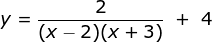### Page 3

#### Question 11 11. Write the following as an exponential function: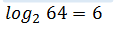#### Question 12 12. What is the global minimum for the function y=f(x) below between x=0 and x=2?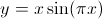#### Question 13 13. In the pictured graph of f(x) = -2x^2 + 4x - 2, for which values of x is the function decreasing?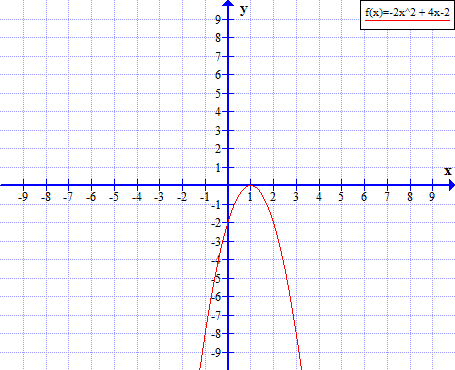#### Question 15 15. In the pictured graph of f(x) = x^2 + 6x, for which values of x is the function increasing?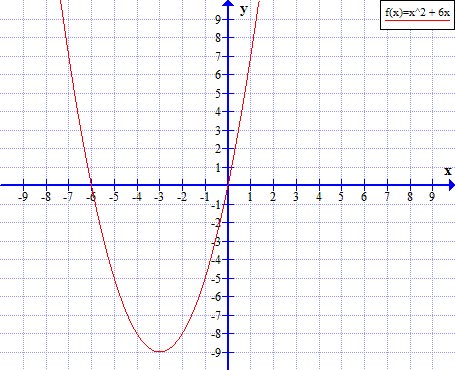### Page 4

#### Question 16 16. Given the function below, evaluate g(7).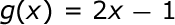### Page 6

#### Question 27 27. In the pictured graph of f(x) = x^2 - 4x, for which values of x is f(x) > 0?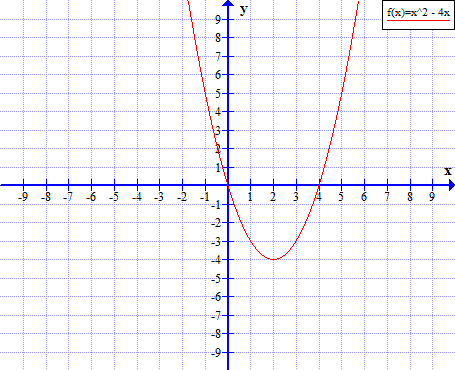#### Question 29 29. Which equation matches the following graph?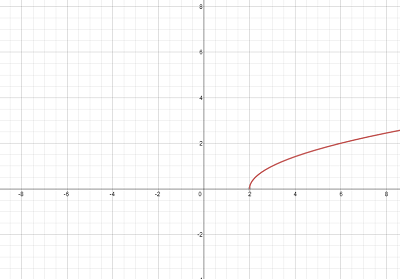#### Basics of Functions Chapter Exam Instructions

Choose your answers to the questions and click 'Next' to see the next set of questions. You can skip questions if you would like and come back to them later with the yellow "Go To First Skipped Question" button. When you have completed the practice exam, a green submit button will appear. Click it to see your results. Good luck!

Support